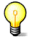# Cauchy problems for a system of non-linear equations and for the non-linear Schrodinger equation

Bước tới: chuyển hướng, tìm kiếm
 Cauchy problems for a system of non-linear equations and for the non-linear Schrodinger equation ' Tạp chí Inverse Problems 1994 ; 10 ():415 - 429 Tác giả Phạm Lợi Vũ Nơi thực hiện Inst. of Mech., Nat. Centre for Sci. Res. of Vietnam, Hanoi, Viet Nam Từ khóa DOI   URL  PDF

## Tóm tắt

The paper deals with Cauchy problems for the system of non-linear equations on a half-line ic1t=c1xx-2c12c2 -ic2t=c2xx-2c1c22 0<or=x< infinity . The first problem for the non-linear Schrodinger equation (NLS) is obtained from the system when c1=C2. In this case the boundary problem associated with this NLS does not have a discrete spectrum, and the solution of the NLS is found uniquely from the given initial condition. Further we show a remarkable class of matrix potentials, in which the inverse scattering problem associated with the system can be solved exactly. In the case that the given scattering data for consist of only two simple poles the exact soliton solution of the system is presented. This happens if and only if the time evolution of the scattering data for obeys some time evolution equations. In this case the Cauchy problem for the NLS, which is obtained when c1=-c2, is solved exactly.